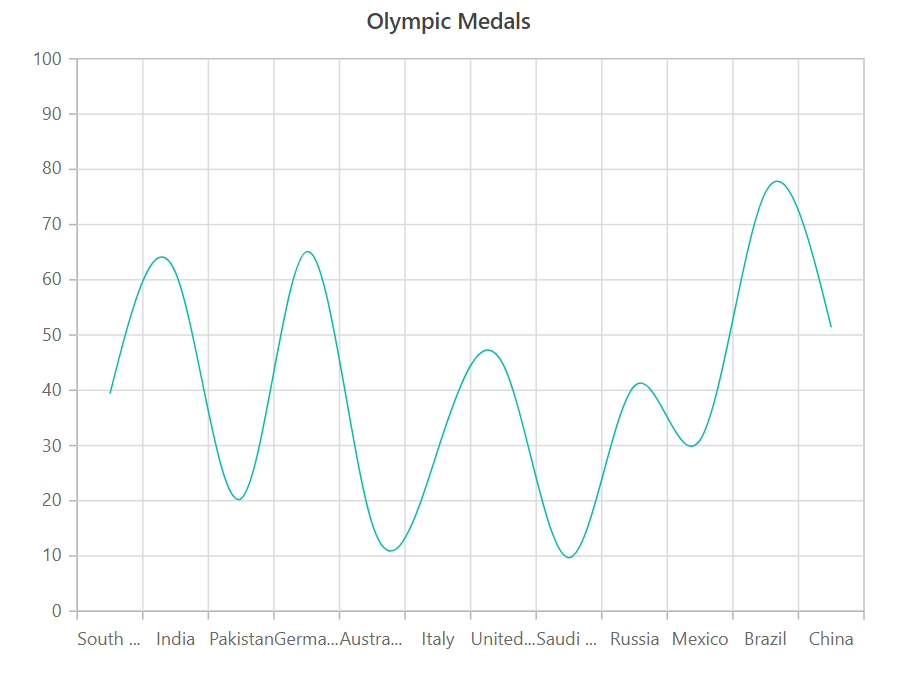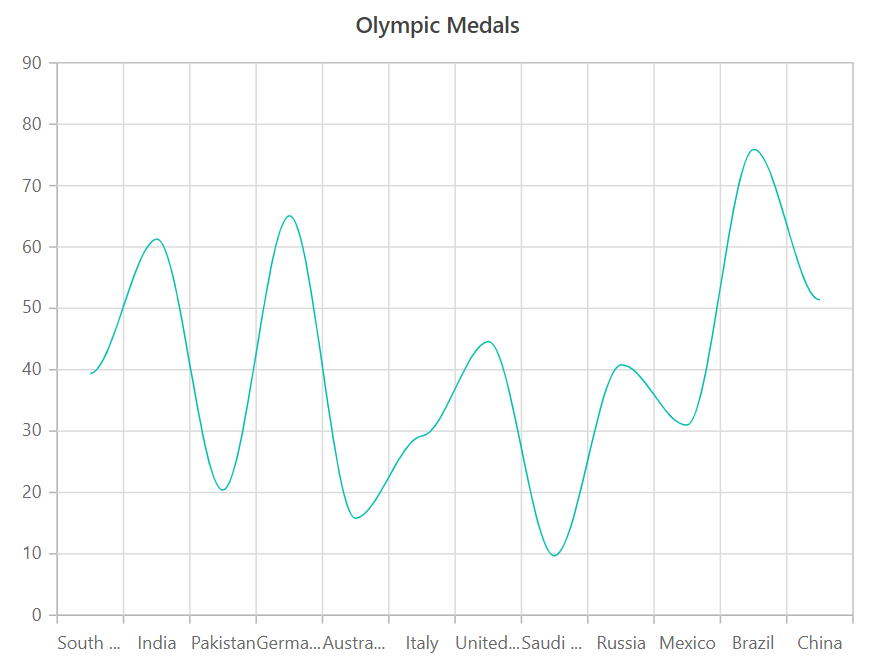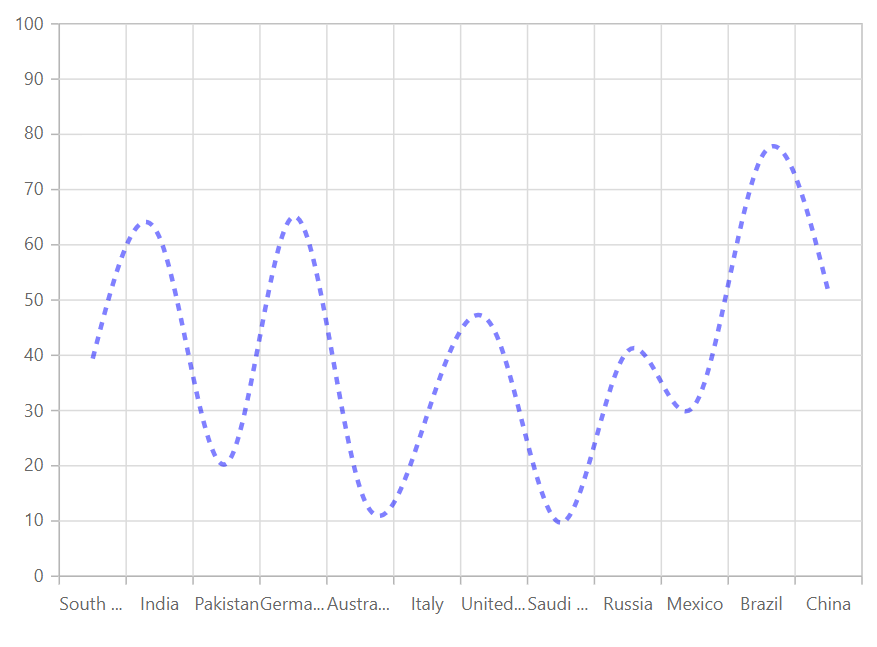Search results

# Spline in Blazor Chart component

08 Apr 2021 / 3 minutes to read

## Spline

This chart connects the data points with smooth curves. To render a spline series, use series `Type` as `Spline`.

spline.razor
``````@using Syncfusion.Blazor.Charts

<SfChart Title="Olympic Medals">
<ChartPrimaryXAxis ValueType="Syncfusion.Blazor.Charts.ValueType.Category"></ChartPrimaryXAxis>
<ChartSeriesCollection>
<ChartSeries DataSource="@MedalDetails" XName="X" YName="Y" Type="ChartSeriesType.Spline">
</ChartSeries>
</ChartSeriesCollection>
</SfChart>

@code{
public class ChartData
{
public string X { get; set; }
public double Y { get; set; }
}
public List<ChartData> MedalDetails = new List<ChartData>
{
new ChartData { X= "South Korea", Y= 39.4 },
new ChartData { X= "India", Y= 61.3 },
new ChartData { X= "Pakistan", Y= 20.4 },
new ChartData { X= "Germany", Y= 65.1 },
new ChartData { X= "Australia", Y= 15.8 },
new ChartData { X= "Italy", Y= 29.2 },
new ChartData { X= "United Kingdom", Y= 44.6 },
new ChartData { X= "Saudi Arabia", Y= 9.7 },
new ChartData { X= "Russia", Y= 40.8 },
new ChartData { X= "Mexico", Y= 31 },
new ChartData { X= "Brazil", Y= 75.9 },
new ChartData { X= "China", Y= 51.4 }
};
}``````Note: You can refer to our `Blazor Spline Chart` feature tour page to know about its other groundbreaking feature representations. You can also explore our `Blazor Spline Chart Example` to know how to connects the data points with smooth curves.

## SplineType

You can configure the spline type using `SplineType` property. The spline types are `Clamped`, `Cardinal` , `Monotonic` and `Natural`.

``````@using Syncfusion.Blazor.Charts

<SfChart Title="Olympic Medals" Width="60%">
<ChartPrimaryXAxis ValueType="Syncfusion.Blazor.Charts.ValueType.Category"></ChartPrimaryXAxis>

<ChartSeriesCollection>
<ChartSeries DataSource="@MedalDetails" XName="X" YName="Y" Type="ChartSeriesType.Spline" SplineType="SplineType.Cardinal">
</ChartSeries>
</ChartSeriesCollection>
</SfChart>

@code{
public class ChartData
{
public string X;
public double Y;
}
public List<ChartData> MedalDetails = new List<ChartData>
{
new ChartData { X= "South Korea", Y= 39.4 },
new ChartData { X= "India", Y= 61.3 },
new ChartData { X= "Pakistan", Y= 20.4 },
new ChartData { X= "Germany", Y= 65.1 },
new ChartData { X= "Australia", Y= 15.8 },
new ChartData { X= "Italy", Y= 29.2 },
new ChartData { X= "United Kingdom", Y= 44.6 },
new ChartData { X= "Saudi Arabia", Y= 9.7 },
new ChartData { X= "Russia", Y= 40.8 },
new ChartData { X= "Mexico", Y= 31 },
new ChartData { X= "Brazil", Y= 75.9 },
new ChartData { X= "China", Y= 51.4 }
};
}``````## Customization

You can use the following properties to customize the spline series.

``````@using Syncfusion.Blazor.Charts

<SfChart Title="Olympic Medals" Width="60%">
<ChartPrimaryXAxis ValueType="Syncfusion.Blazor.Charts.ValueType.Category"></ChartPrimaryXAxis>

<ChartSeriesCollection>
<ChartSeries DataSource="@MedalDetails" XName="X" YName="Y" Width="3" Opacity="0.5"
DashArray="5,5" Fill="blue" Type="ChartSeriesType.Spline">
</ChartSeries>
</ChartSeriesCollection>
</SfChart>

@code{
public class ChartData
{
public string X;
public double Y;
}
public List<ChartData> MedalDetails = new List<ChartData>
{
new ChartData { X= "South Korea", Y= 39.4 },
new ChartData { X= "India", Y= 61.3 },
new ChartData { X= "Pakistan", Y= 20.4 },
new ChartData { X= "Germany", Y= 65.1 },
new ChartData { X= "Australia", Y= 15.8 },
new ChartData { X= "Italy", Y= 29.2 },
new ChartData { X= "United Kingdom", Y= 44.6 },
new ChartData { X= "Saudi Arabia", Y= 9.7 },
new ChartData { X= "Russia", Y= 40.8 },
new ChartData { X= "Mexico", Y= 31 },
new ChartData { X= "Brazil", Y= 75.9 },
new ChartData { X= "China", Y= 51.4 }
};
}``````Note: You can refer to our `Blazor Charts` feature tour page for its groundbreaking feature representations. You can also explore our `Blazor Chart example` to knows various chart types and how to represent time-dependent data, showing trends in data at equal intervals.

Contents
Contents